# 呆呆带你手撸一个思维导图-基础篇## 前言

• 基础篇：实现思维导图的一种方案
• 实战篇：`react-topic`项目初步搭建
• 实战篇：连线的具体实现
• 实战篇：能动的导图• 明确目标
• 节点的实现思路
• `svg`及二次贝塞尔曲线
• 连线的实现思路

## 1. 明确目标

XMind: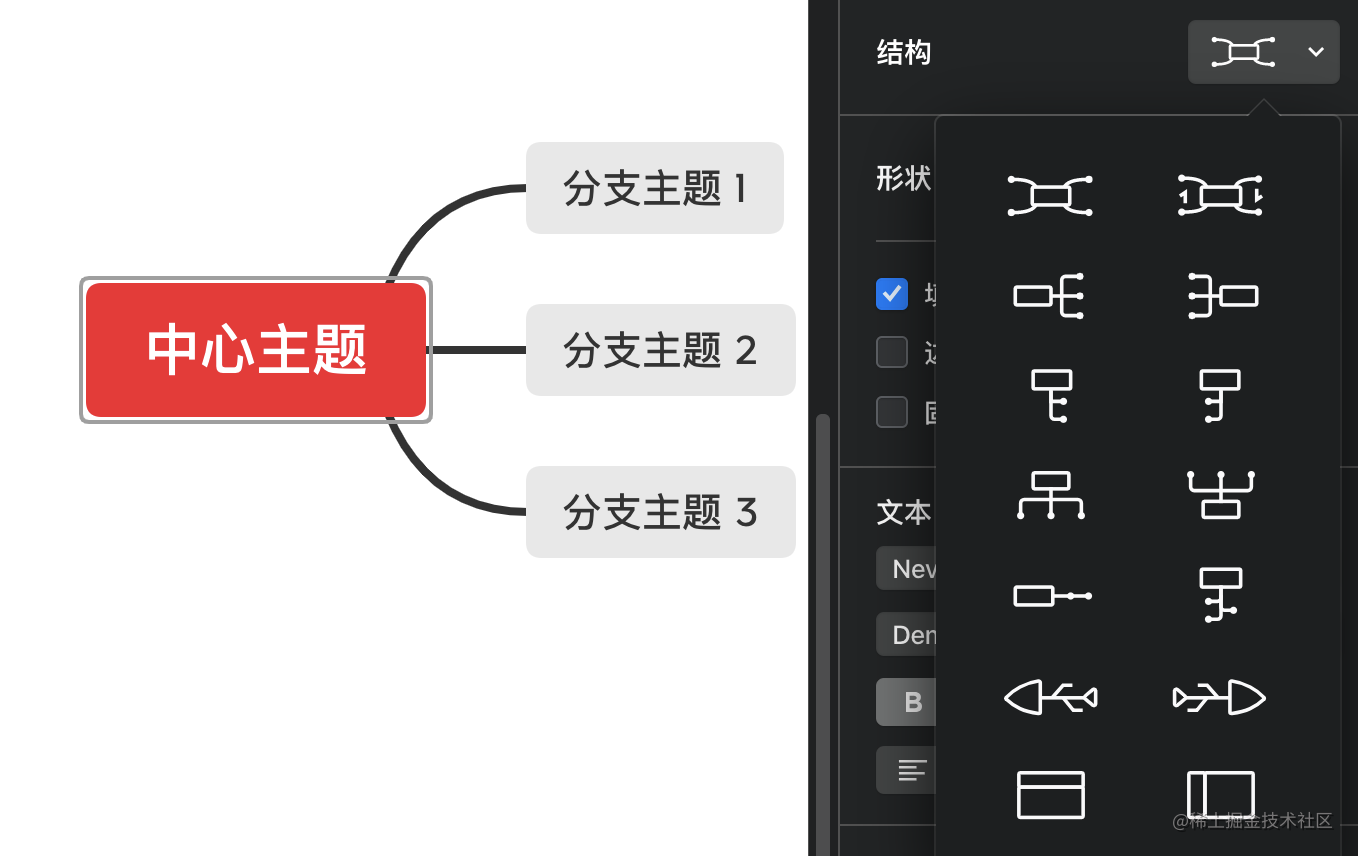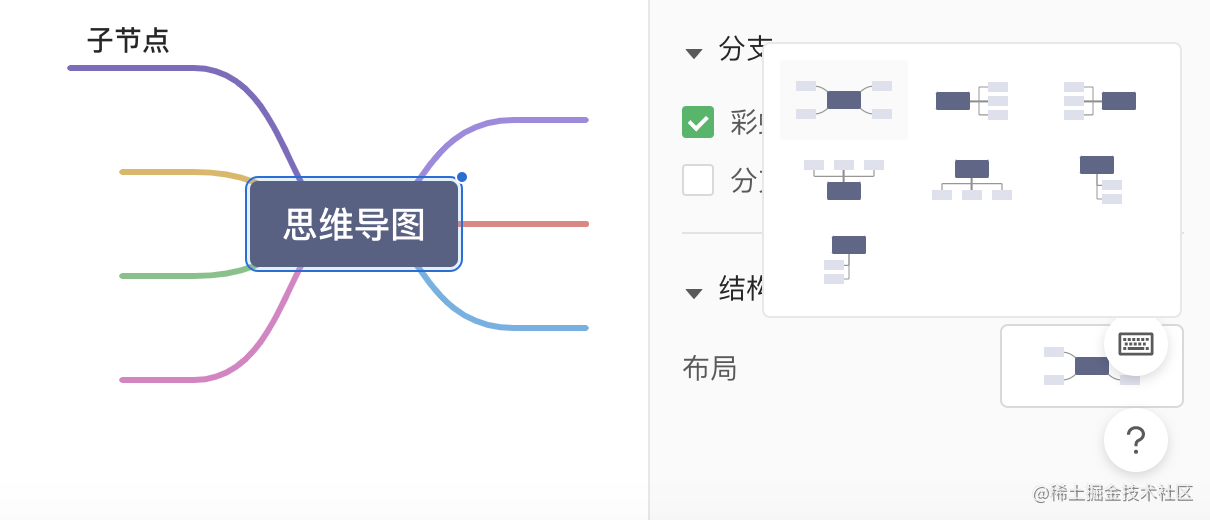processon：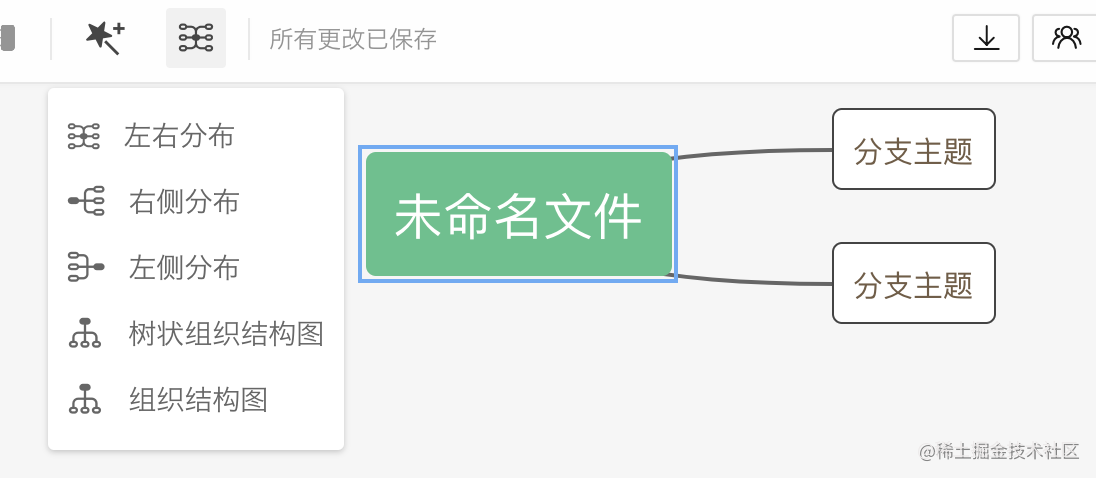• 类型丰富，左右子树布局(我们称为脑图)、组织结构图、鱼骨图等。
• 节点都可以进行拖拽移动改变层级
• ...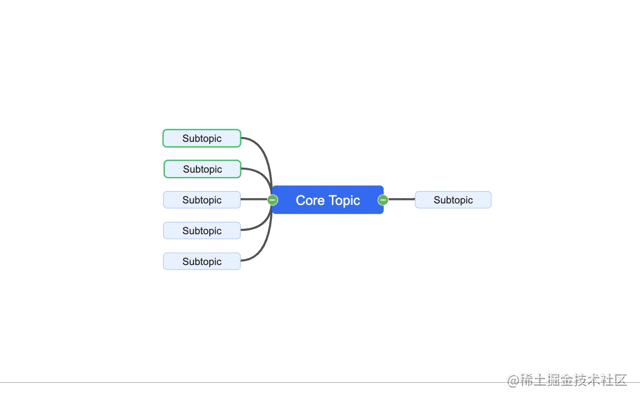## 2. 节点的实现思路

### 2.1 方案选型

• 节点(主题)
• 连线

(嘻嘻，既然说到了这，哎，推一波推一波：《全网最详bpmn教材》)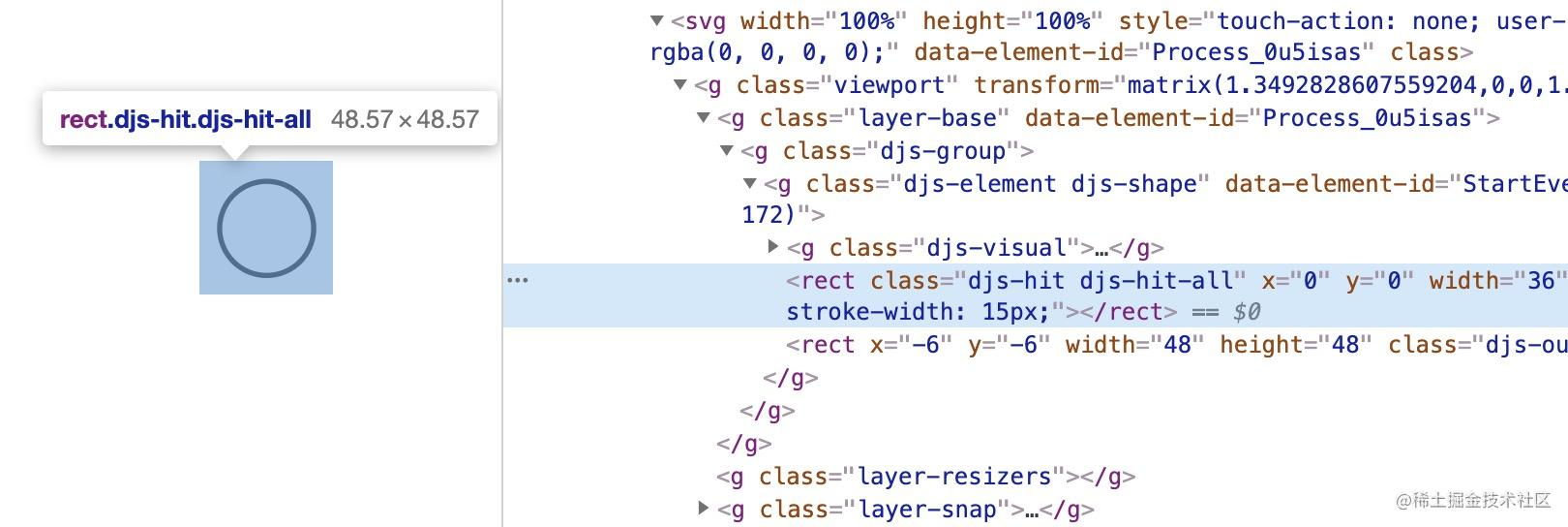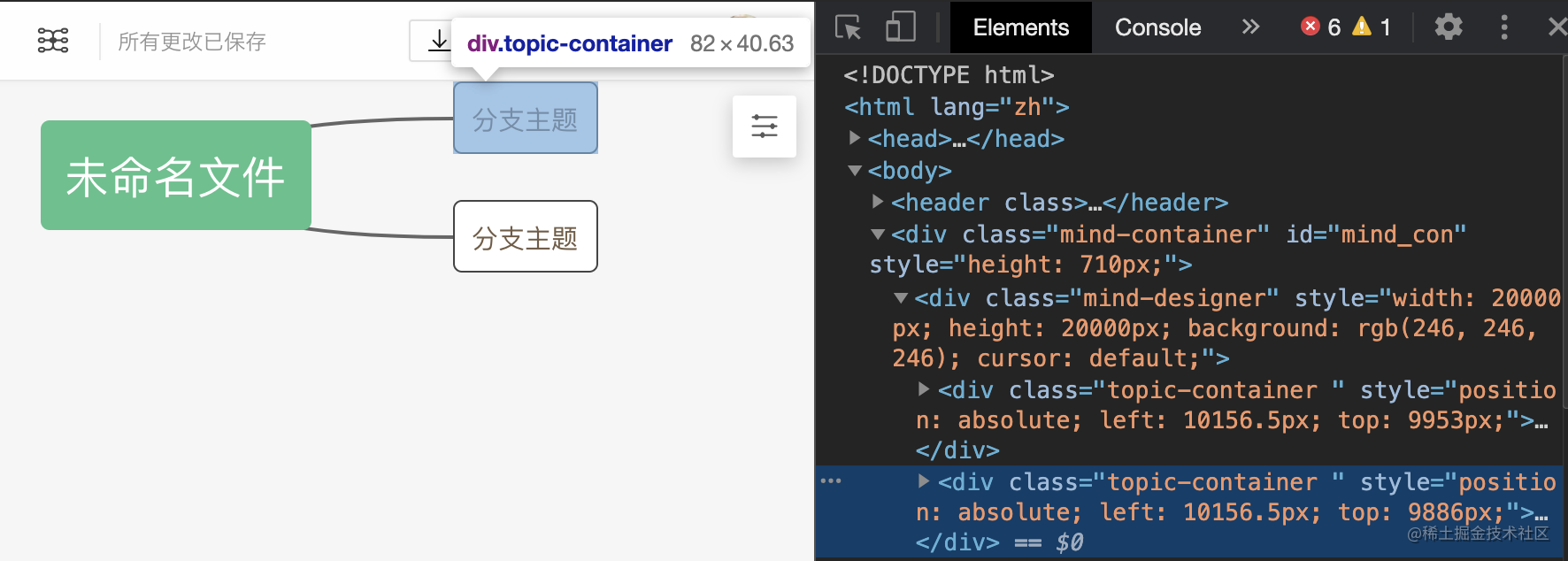### 2.2 DOM元素 + flex布局

• 左子树
• 根节点
• 右子树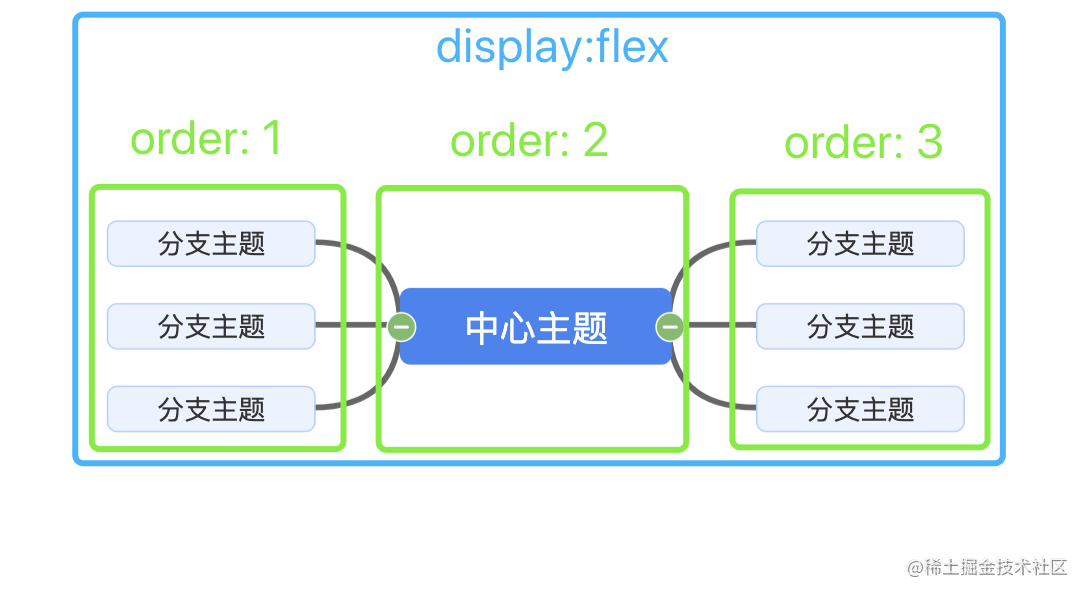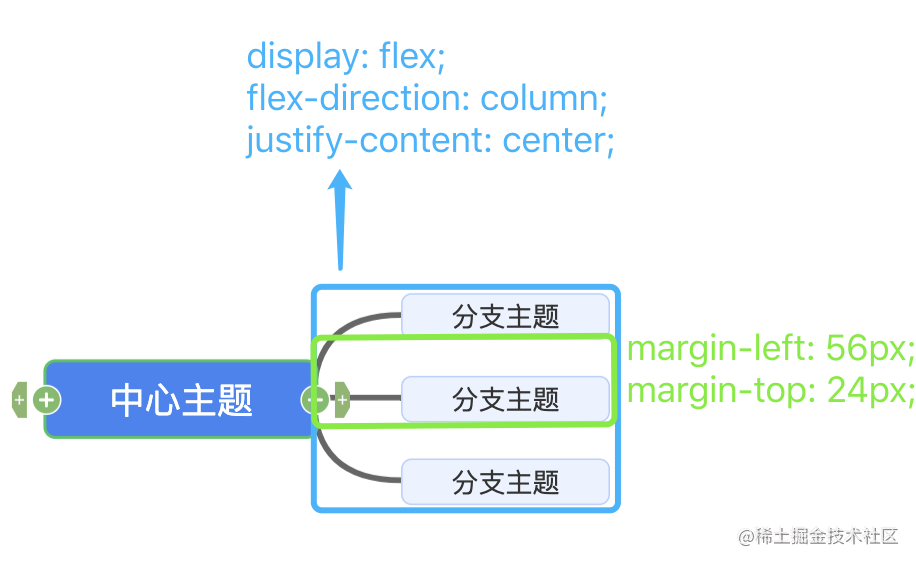html代码：

``````<div class="mindMap">
<div class="node rootNode">根节点</div>
<div class="node subTree left">
<div class="treeNode">子节点</div>
<div class="treeNode">子节点</div>
<div class="treeNode">子节点</div>
</div>
<div class="node subTree right">
<div class="treeNode">子节点</div>
<div class="treeNode">子节点</div>
<div class="treeNode">子节点</div>
</div>
</div>

css代码：

``````.mindMap {
display: flex;
align-items: center;
}
.node {
width: 200px;
background: rgb(192 214 255);
text-align: center;
color: #fff;
}
.rootNode {
order: 2;
background: #65bfff;
height: 50px;
}
.left {
order: 1;
}
.right {
order: 3;
}
.subTree {
display: flex;
flex-direction: column;
justify-content: center;
}
.treeNode {
background-color: #ff6969;
}
.left .treeNode {
margin-right: 56px;
}
.right .treeNode {
margin-left: 56px;
}
.treeNode:not(:first-child) {
margin-top: 24px;
}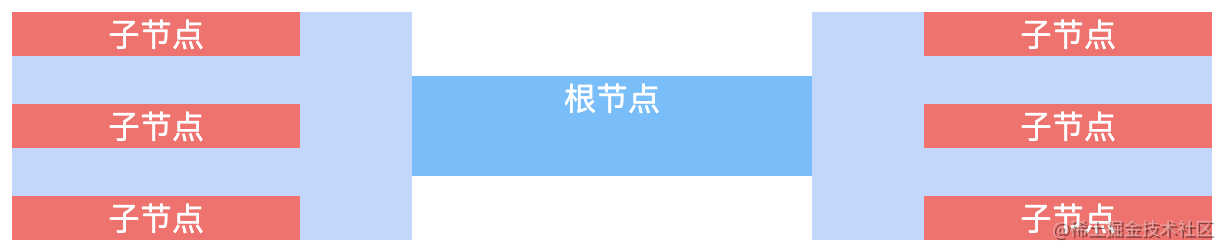## 3. svg及二次贝塞尔曲线

### 3.1 svg简介

`svg`为可缩放矢量图形，它使用`XML`格式来定义图像。我们可以像使用一个`div`标签一样直接在代码中使用：

``````<svg width="300" height="300">
<line x1="0" y1="0" x2="200" y2="0" />
</svg>

• `fill`：填充色
• `stroke`：描边色
• `stroke-width`：边框宽度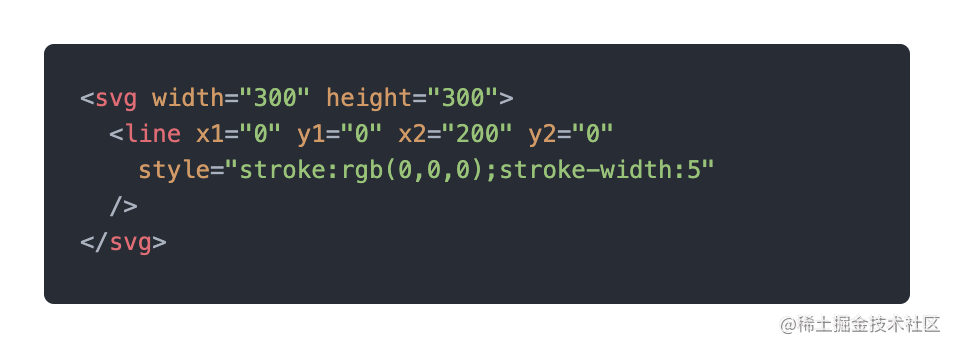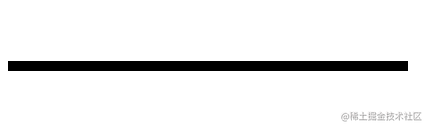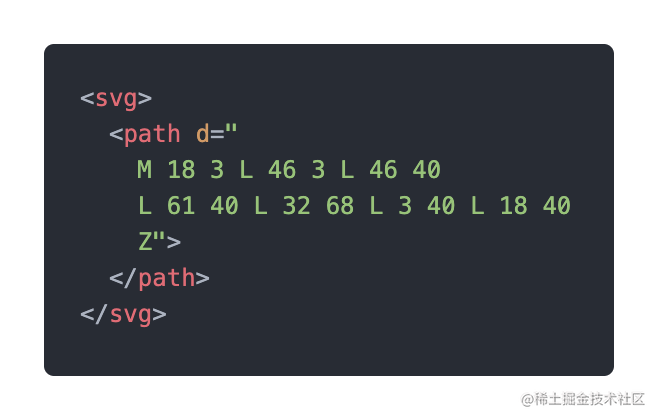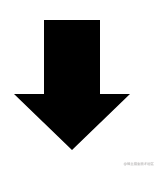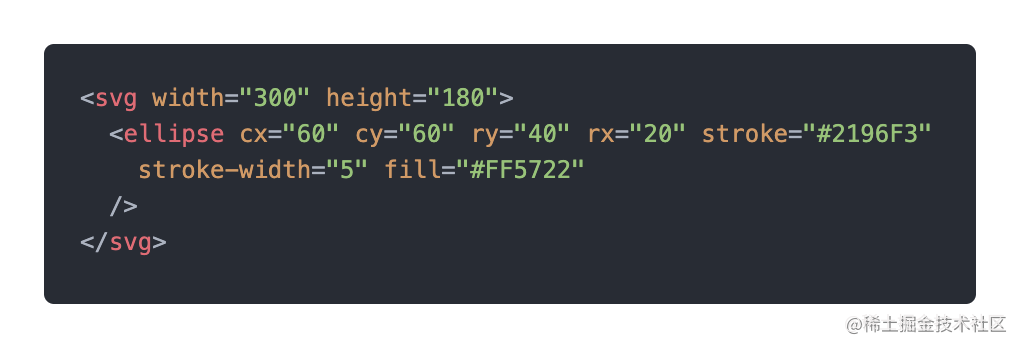### 3.2 path元素

`path`元素可用来创建线条、曲线、弧形等等。一个path元素形状的定义是依靠于属性 `d`, 属性`d` 的值是一个 `“命令 + 参数”`的序列。

``````<svg>
<path d="M10 10 L90 10"
stroke="rgb(0,0,0)" stroke-width="5"
></path>
</svg>

• 移动到坐标为 (10, 10) 的点；
• 绘制一条线到坐标为 (90, 10) 的位置。

(`M: Move to` 移动到某个坐标 ;    `L: Line to` 在某两个坐标之间画一条线)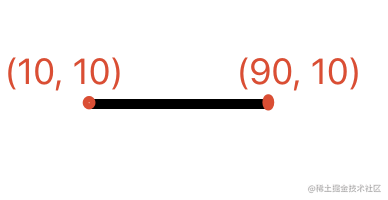### 3.3 二次贝塞尔曲线Q

• 三次贝塞尔曲线`C`
• 二次贝塞尔曲线`Q`

• 起点坐标
• 控制点坐标
• 终点坐标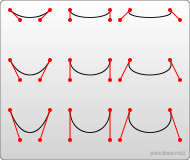``````<svg>
<path
path d="M10 80 Q 95 10 180 80"
stroke="black" fill="transparent"
></path>
</svg>

• 移动到坐标为 (10, 80) 的点；
• 控制点的坐标为 (95, 10)；
• 终点的坐标为 (180, 80)；
• 根据控制点得到起点和终点的斜率并在它们两之间绘制一条曲线。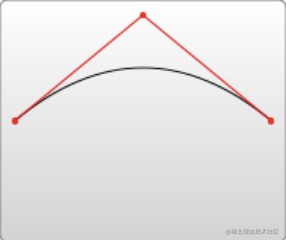## 4. 连线的实现思路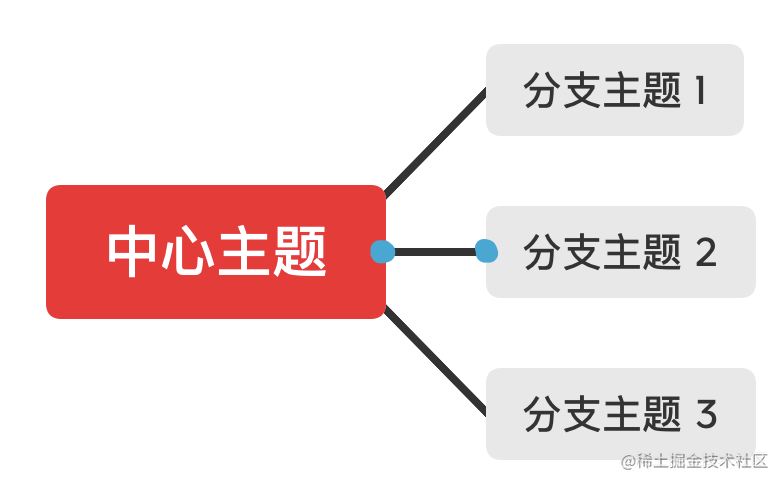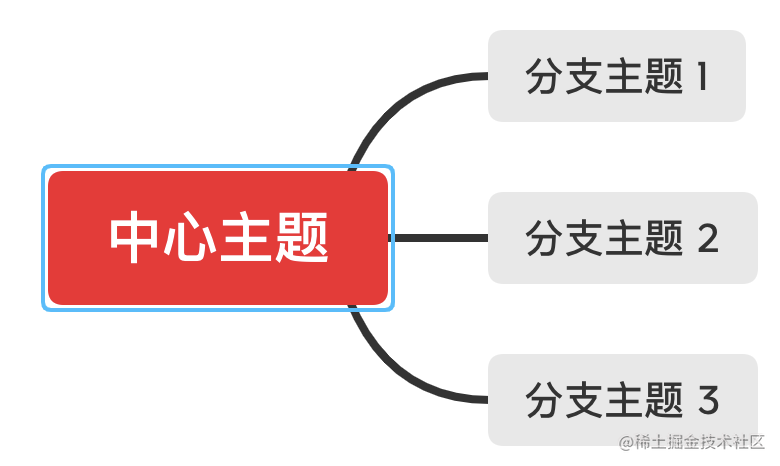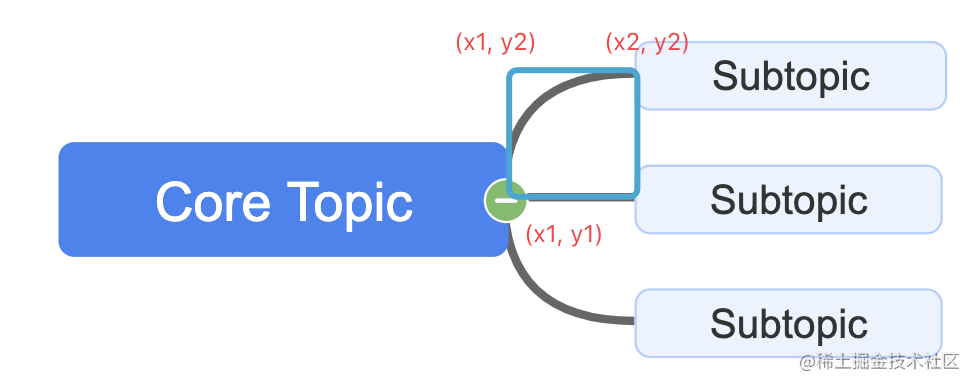• 起点：(x1, y1)     => (100, 60)
• 控制点：(x1, y2)  => (100, 10)
• 终点：(x2, y2)     => (150, 10)

(这里的坐标，是以思维导图整体最左上角为坐标轴的起点)

``````<svg>
<path
d="M100 60 Q 100 10 150 10"
fill="transparent"
stroke="rgb(102, 102, 102)"
stroke-width="4"
></path>
</svg>